# 前言

OctaveNet网络paper是《Drop an Octave: Reducing Spatial Redundancy in Convolutional Neural Networks with Octave Convolution》，是CVPR2019中的一篇论文。
OctaveNet是一个用于ImageNet Classfication任务的backbone结构。这篇论文提出了一种新型的卷积结构，或者叫做卷积模块，叫做 Octave Convolution。
Octave Convolution号称是一种可以无缝嵌入到任何已有backbone中的模块，简单好用，能有效降低已有模型的计算量并带来小幅的性能提升，听起来还是让人非常兴奋的。

# 从频域的角度理解图像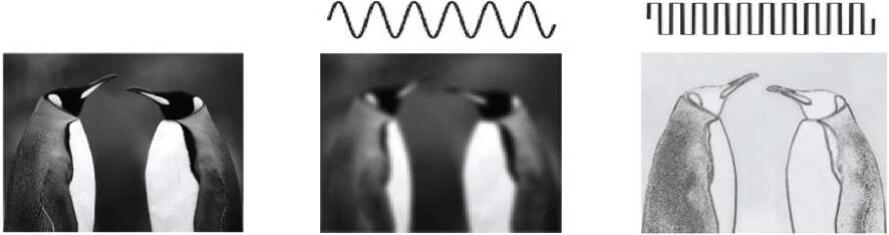# 特征图的高频与低频表示

“下采样”的scale，采用的是2的幂次，而目前文章只讨论了 2 1 2^{1} 次幂的情况，说白了就是特征图的长宽都缩小了2，就像下面这张图：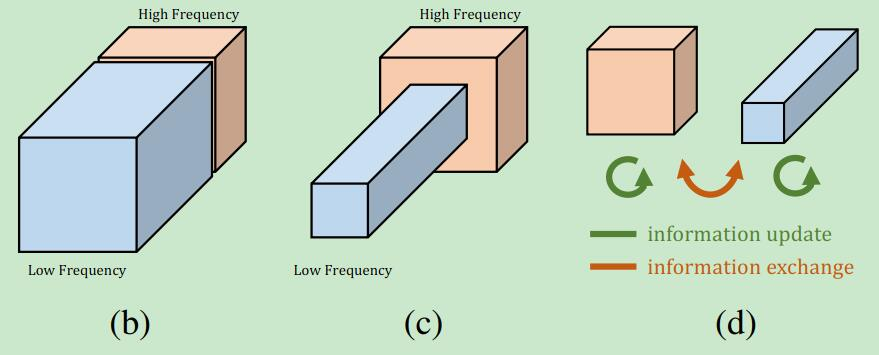# Octave Convolution

## Octave Convolution原理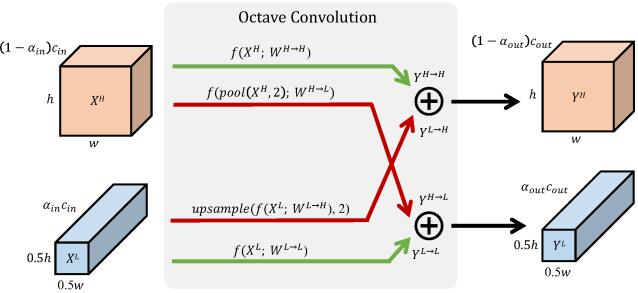（1）高频部分直接卷积： f ( X H ) f\left ( X^{H}\right ) ，即高频到高频的卷积，输出通道数 ( 1 − a o u t ) c o u t \left ( 1- a_{out}\right )c_{out}
（2）高频部分先做下采样再卷积，这里的下采样是 p o o l ( X H , 2 ) pool\left ( X^{H},2\right ) ，然后 f ( p o o l ( X H , 2 ) ) f\left ( pool\left ( X^{H},2\right )\right) ，即高频到低频的卷积，输出通道数 a o u t c o u t a_{out}c_{out}
（3）低频部分直接卷积后做上采样： f ( X L ) f\left ( X^{L}\right ) ，这里的 u p s a m p l e ( f ( X L ) ) upsample\left ( f\left ( X^{L}\right )\right ) 所用的上采样方法我们后面再说，即低频到高频的卷积，输出通道数 ( 1 − a o u t ) c o u t \left ( 1- a_{out}\right )c_{out}
（4）低频部分直接卷积： f ( X L ) f\left ( X^{L}\right ) ，即低频到低频的卷积，输出通道数 a o u t c o u t a_{out}c_{out}

## 上采样和下采样

Octave Convolution的低频域输出可以用下面的公式表示：
Y p , q L = Y p , q L → L + Y p , q H → L Y_{p,q}^{L}=Y_{p,q}^{L\rightarrow L}+Y_{p,q}^{H\rightarrow L}
Y p , q L → L = ∑ i , j ∈ N k W i + k − 1 2 , j + k − 1 2 L → L X p + i , q + j L Y_{p,q}^{L\rightarrow L}=\sum_{i,j\in N{_{k}}}W_{i+\frac{k-1}{2},j+\frac{k-1}{2}}^{L\rightarrow L}X_{p+i,q+j}^{L}
Y p , q H → L = ∑ i , j ∈ N k W i + k − 1 2 , j + k − 1 2 H → L X 2 ∗ p + i , 2 ∗ q + j H Y_{p,q}^{H\rightarrow L}=\sum_{i,j\in N{_{k}}}W_{i+\frac{k-1}{2},j+\frac{k-1}{2}}^{H\rightarrow L}X_{2*p+i, 2*q+j}^{H}

Octave Convolution的高频域输出可以用下面的公式表示：
Y p , q H = Y p , q H → H + Y p , q L → H Y_{p,q}^{H}=Y_{p,q}^{H\rightarrow H}+Y_{p,q}^{L\rightarrow H}
Y p , q H → H = ∑ i , j ∈ N k W i + k − 1 2 , j + k − 1 2 H → H X p + i , q + j H Y_{p,q}^{H\rightarrow H}=\sum_{i,j\in N{_{k}}}W_{i+\frac{k-1}{2},j+\frac{k-1}{2}}^{H\rightarrow H}X_{p+i,q+j}^{H}
Y p , q L → H = ∑ i , j ∈ N k W i + k − 1 2 , j + k − 1 2 H → L X p 2 + i , q 2 + j L Y_{p,q}^{L\rightarrow H}=\sum_{i,j\in N{_{k}}}W_{i+\frac{k-1}{2},j+\frac{k-1}{2}}^{H\rightarrow L}X_{\frac{p}{2}+i, \frac{q}{2}+j}^{L}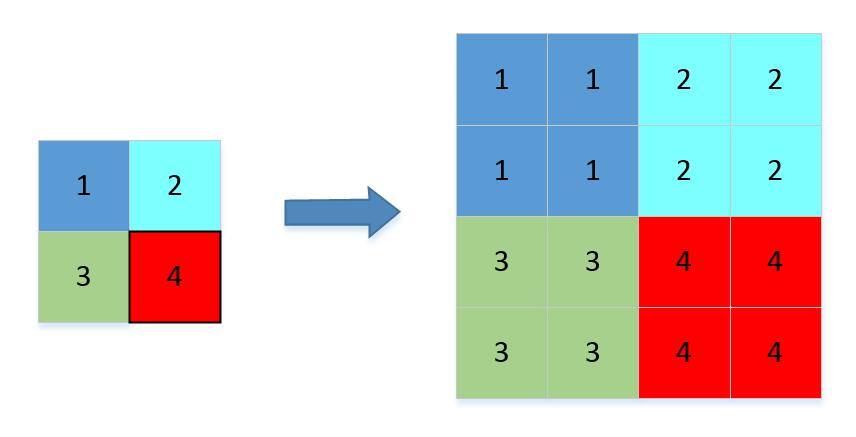## OctaveConv如何减低计算量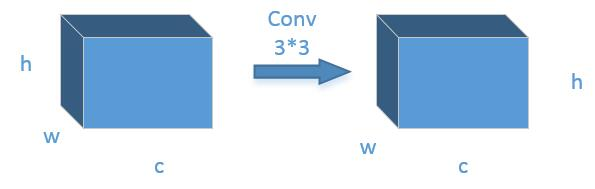C o m p o n c e = ( 3 × 3 + 8 ) × c + c − 1 Comp_{once} = \left ( 3\times3+8\right )\times c + c-1

C o m p b = C o m p o × c × w × h Comp_{b} =Comp_{o}\times c \times w \times h

C o m p b = ( 3 × 3 + 8 ) × c × c × w × h Comp_{b} = \left ( 3\times3+8\right )\times c \times c \times w \times h

C o m p o 1 = ( 3 × 3 + 8 ) × 1 2 c × 1 2 c × w × h = 1 4 C o m p a Comp_{o1} = \left ( 3\times3+8\right )\times \frac{1}{2}c \times \frac{1}{2}c \times w \times h = \frac{1}{4}Comp_{a}

C o m p o 2 = ( 3 × 3 + 8 ) × 1 4 c × 1 4 c × 1 4 w × 1 4 h = 1 16 C o m p a Comp_{o2} = \left ( 3\times3+8\right )\times \frac{1}{4}c \times \frac{1}{4}c \times \frac{1}{4}w \times \frac{1}{4}h = \frac{1}{16}Comp_{a}

C o m p o 3 = ( 3 × 3 + 8 ) × 1 4 c × 1 4 c × 1 4 w × 1 4 h = 1 16 C o m p a Comp_{o3} = \left ( 3\times3+8\right )\times \frac{1}{4}c \times \frac{1}{4}c \times \frac{1}{4}w \times \frac{1}{4}h = \frac{1}{16}Comp_{a}

C o m p o 4 = ( 3 × 3 + 8 ) × 1 4 c × 1 4 c × 1 4 w × 1 4 h = 1 16 C o m p a Comp_{o4} = \left ( 3\times3+8\right )\times \frac{1}{4}c \times \frac{1}{4}c \times \frac{1}{4}w \times \frac{1}{4}h = \frac{1}{16}Comp_{a}

C o m p o = = 7 16 C o m p a Comp_{o} = = \frac{7}{16}Comp_{a}

# OctaveConv的Caffe实现

## octave_upsample_layer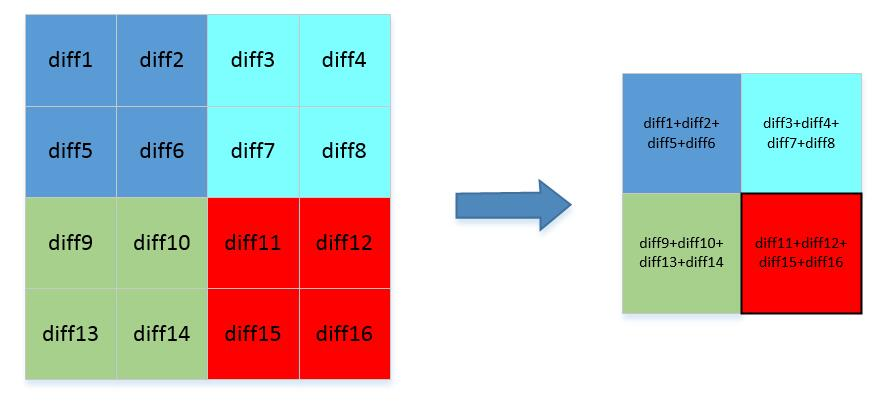## 自定义层注册

message LayerParameter {
optional OctaveUpsampleParameter octaveupsample_param = 最后一个ID;
}


message OctaveUpsampleParameter {
// DEPRECATED. No need to specify upsampling scale factors when
// exact output shape is given by upsample_h, upsample_w parameters.
optional uint32 scale = 1 [default = 2];
// DEPRECATED. No need to specify upsampling scale factors when
// exact output shape is given by upsample_h, upsample_w parameters.
optional uint32 scale_h = 2;
// DEPRECATED. No need to specify upsampling scale factors when
// exact output shape is given by upsample_h, upsample_w parameters.
optional uint32 scale_w = 3;
// DEPRECATED. Specify exact output height using upsample_h. This
// parameter only works when scale is 2
optional bool pad_out_h = 4 [default = false];
// DEPRECATED. Specify exact output width using upsample_w. This
// parameter only works when scale is 2
optional bool pad_out_w = 5 [default = false];
optional uint32 upsample_h = 6;
optional uint32 upsample_w = 7;
}


# OctaveConv一定能让网络变快吗？

resnet18的conv19耗时

conv19   forward: 6.9 ms.


resnet18_octave_0.5conv19耗时6.9ms

 conv19_hf                forward: 3 ms.
conv19_lf                 forward: 1.24 ms.


04-193047
04-161万+08-212918
05-13750
08-151388
05-201626
01-031万+
07-252987
11-291023
11-161463
©️2020 CSDN 皮肤主题: Age of Ai 设计师:meimeiellie